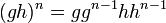# (n-1)th power map is endomorphism taking values in the center implies nth power map is endomorphism

(diff) ← Older revision | Latest revision (diff) | Newer revision → (diff)
Jump to: navigation, search

## Statement

Suppose$G$ is a group and$n$ is an integer such that the power map$x \mapsto x^{n-1}$ is an endomorphism of$G$ and$x^{n-1}$ is in the center of$G$ for all$x$ in$G$. Then, the map$x \mapsto x^n$ is also an endomorphism of$G$.

## Related facts

### Converse

The precise converse is not true, but a partial converse is: nth power map is surjective endomorphism implies (n-1)th power map is endomorphism taking values in the center

## Proof

Given: A group$G$, an integer$n$ such that the map$x \mapsto x^{n-1}$ is an endomorphism taking values in the center.

To prove: The map$x \mapsto x^n$ is an endomorphism of$G$, i.e.,$(gh)^n = g^nh^n$ for all$g,h \in G$.

Proof: We have the following for all$g,h \in G$.

Step no. Assertion/construction Given data used Previous steps used
1$(gh)^n = (gh)(gh)^{n-1}$
2$(gh)^{n-1} = g^{n-1}h^{n-1}$$x \mapsto x^{n-1}$ is an endomorphism
3$(gh)^n = ghg^{n-1}h^{n-1}$ Step (2) plugged into Step (1)
4$(gh)^n = gg^{n-1}hh^{n-1}$$g^{n-1}$ is in the center of$G$, so commutes with$h$ Step (3)
5$(gh)^n = g^nh^n$ Step (4) (simplified)